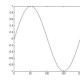# Digital Gal

United States

Top Questions

## Good 1st PDE book for self study

asked Aug 19 '10 at 20:13

## Group where every element is order 2

asked Jan 11 '11 at 1:38

## Applications of Gröbner bases

asked Apr 12 '11 at 0:10

## If $\sum_{n=1}^{\infty} a_{n}^{3}$ converges does $\sum_{n=1}^{\infty} \frac{a_{n}}{n}$ converge?

asked Aug 6 '10 at 22:28

## When does the product of two polynomials = $x^{k}$?

asked Aug 26 '10 at 14:45

## Risk Neutral Probability

asked Oct 24 '13 at 20:18